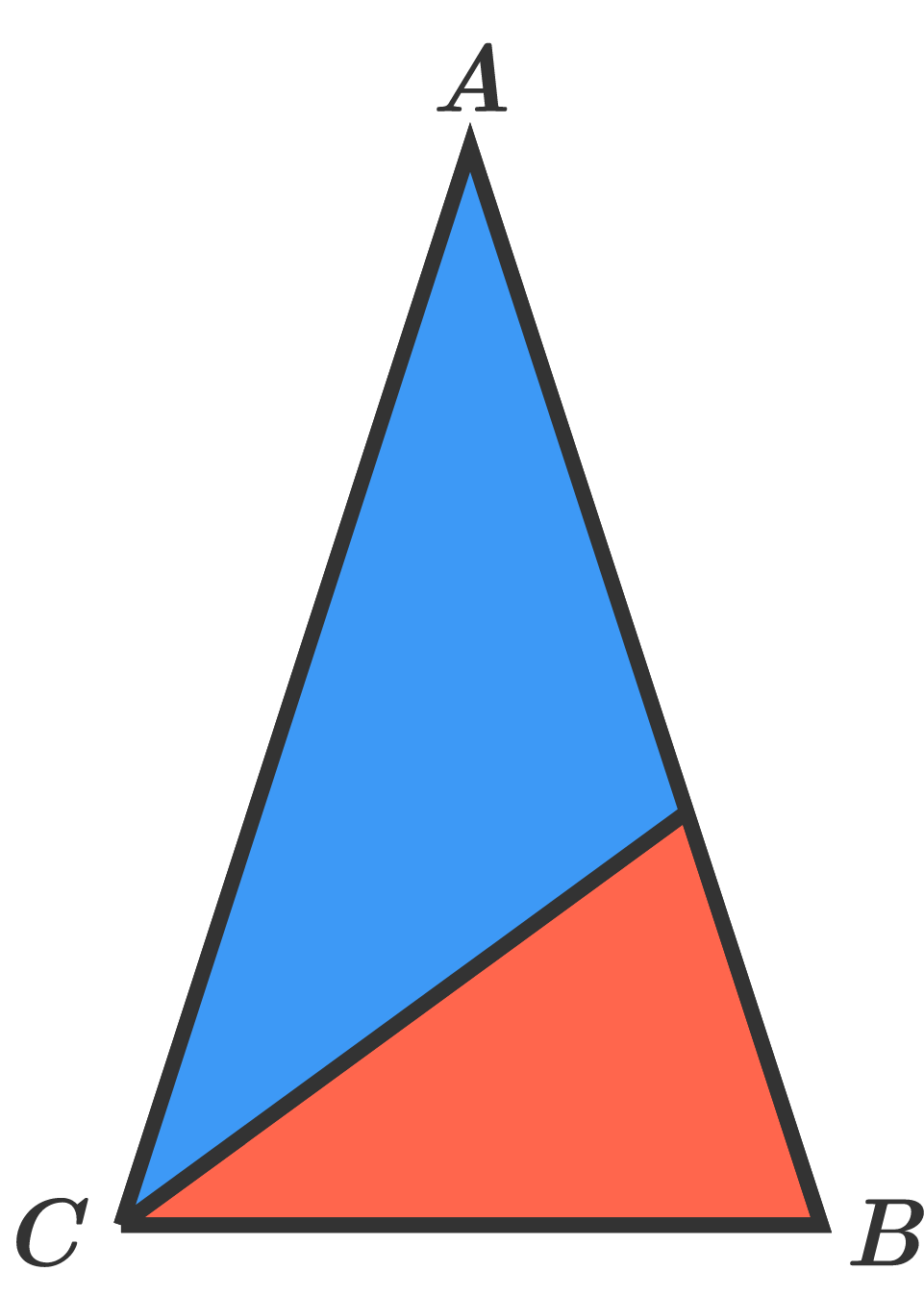# One to Two,Isosceles to Isosceles

Geometry Level 3One possible way to draw $ABC$ and split it into two isosceles triangles is shown here.

An isosceles triangle $ABC$ $($with $\angle B=\angle C)$ is cut into two smaller isosceles triangles (which don't have to be identical).

How many different possible angles $\angle A$ are there?

×

Problem Loading...

Note Loading...

Set Loading...